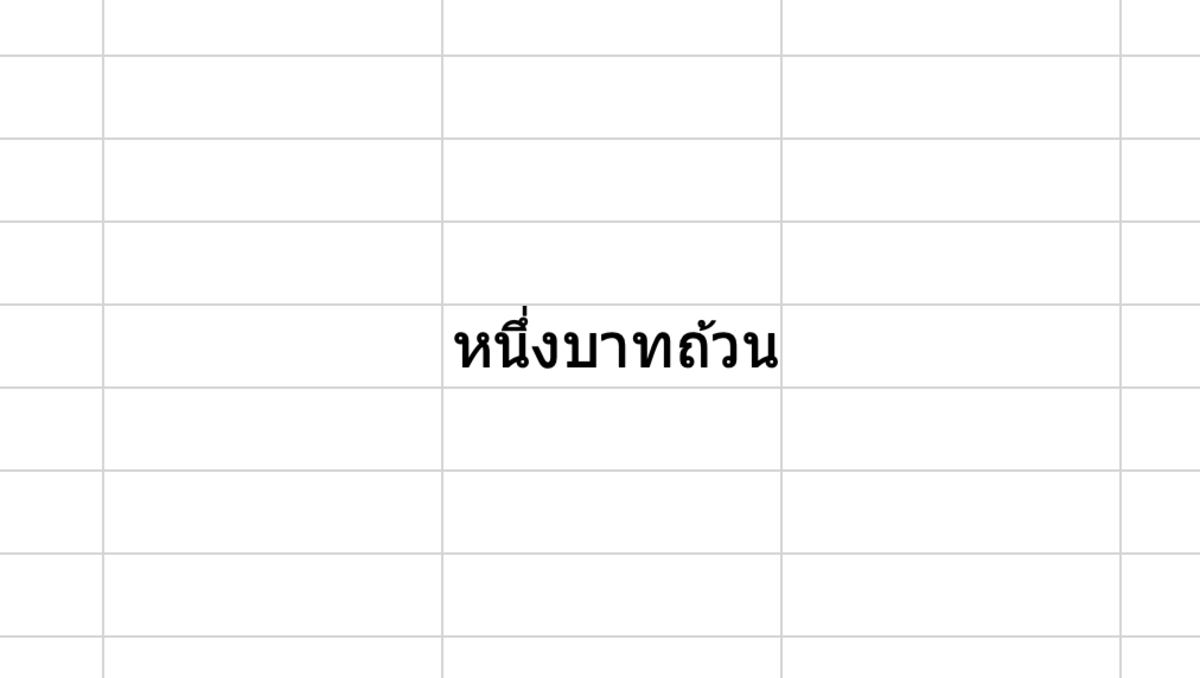# How to Use the BAHTTEXT Function in Excel for Mac

Joshua earned an MBA from USF and he writes mostly about software and technology.

## The Purpose of the BAHTTEXT Function

The BAHTTEXT function gives an Excel user the ability to convert numbers into Thai text that describes the amount in Thai currency. Baht is the official currency used in Thailand. For instance, if you need to translate 1 to 1 Thai baht (หนึ่งบาทถ้วน) or any other quantity of Thai currency, this function would be purposeful.The BAHTTEXT function gives an Excel user the ability to convert numbers into Thai text.Joshua Crowder

## The BAHTTEXT Function Syntax

The BAHTTEXT function needs to be inputted into a cell like a formula for it work. To manually add this function the following steps need to be taken:

1. A cell needs clicked type "=BAHTTEXT(".
2. After the open parenthesis add a number followed by a closed parenthesis.
3. After the formula is created the formula can be entered.

The simple syntax of the BAHTTEXT function is shown below.

=BAHTTEXT(Number)

Number - The number that you would like to convert to Thai text. This can also be a cell reference to a cell containing a number or formula that results in a number.

## BAHTTEXT Examples

Please see the examples below for converting the numbers 8,10,20,40 and 80. Be aware that you may also use cell references and formulas as stated above.

Cell reference Example

=BAHTTEXT(A5)

Formula Example

=BAHTTEXT((A5+4)/A7)

If you are using a cell reference with a cell that will not always contain a value, you may want to consider using the IFERROR function as well.

=IFERROR(BAHTTEXT(A5),"")

Scroll to Continue

## Inserting the BAHTTEXT Function

The BAHTTEXT function can be inserted into a cell by first clicking into a cell followed by clicking on the formula tab. Next, click on the text button and choose the BAHTTEXT function from the list.

Here in the formula build you can add a number to see what results will output. After choosing reviewing the result click done so the result will show in the previously chosen cell.

Microsoft. (n.d.). BAHTTEXT function. Retrieved July 21, 2022, from https://support.microsoft.com/en-us/office/bahttext-function-5ba4d0b4-abd3-4325-8d22-7a92d59aab9c

Wikipedia. (n.d.). Thai baht. Retrieved July 21, 2022, from https://en.wikipedia.org/wiki/Thai_baht

How to Use the COUNT Function in Excel

How to Use the AVERAGE Function in Excel

How to Use the ABS Function in Excel

How to Remove Errors in Excel With the IFERROR Function

How to Use the VLOOKUP Function in Excel

Converting Measurement Units: Using the CONVERT Function

To learn more about using functions in Excel I recommend The Excel 2019 Bible book. I have been using the Excel Bible for years to improve my understanding of all aspects of this Microsoft product.

This content is accurate and true to the best of the author’s knowledge and is not meant to substitute for formal and individualized advice from a qualified professional.